# Basic Tutorial Lesson 12: Analyzing Basic Mixed-Mode Circuits with Logic Gates

 Tutorial Project: Analyzing Basic Mixed-Mode Circuits with Logic Gates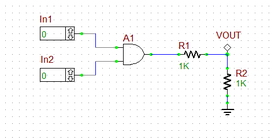Objective: In this project, the basic concepts of analog-to-digital and digital-to-analog conversion in mixed-mode circuits are examined. Concepts/Features: Mixed-Mode Circuit Logic Gates Analog Voltage DAC Bridge ADC Bridge Live Simulation Minimum Version Required: All versions

## What You Will Learn

In this tutorial you will learn how to combine analog and digital devices in the same circuit and perform a mixed-mode simulation of your circuit. You will use DAC and ADC conversion bridges to control the analog voltage levels corresponding to the high and low binary states.

## From Digital Devices to Analog Voltages

RF.Spice A/D allows you to mix digital and analog devices in the same circuit. To simulate the resulting circuit, the program places implicit A/D or D/A converters in your circuit behind the scenes. By default, it is assumed that the "1" or "High" digital state corresponds to a +5V analog voltage, while the "0" or "Low" digital state corresponds to a 0V analog voltage. You can change these conversion values depending on the type of digital device technology you are using in your circuit. This can be done from the SPICE Simulation Options dialog. You can access this dialog from the Simulate Menu. Under the "Mixed Mode" tab of this dialog, you will see two parameters "bb_daOutLow" and "bb_daOutHigh" in the Parameters table with default values of 0 and 5, respectively. To change these values, click on the parameter value in the rightmost column of the table to highlight it and then type in a new value.The SPICE Simulation Options dialog of RF.Spice A/D.

## Testing a Simple AND Gate

The following is a list of parts needed for this part of the tutorial lesson:

Part Name Part Type Part Value
In1 Digital Input 1-bit
In2 Digital Input 1-bit
A1 Generic AND Gate Defaults
R1 - R2 Resistor 1k
VOUT Voltage Probe Marker N/A

As a first step, let's try out a simple AND gate. Place and connect the parts as shown in the figure below. The two 1k resistors form a voltage divider. The voltage probe marker "VOUT" would provide half the voltage at the output of the AND gate.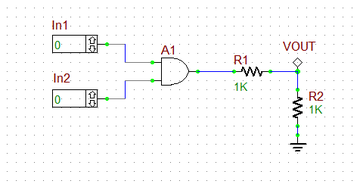A simple AND gate with an analog voltage divider at its output.

Run a live simulation of this circuit and enable circuit animation by clicking the Show Voltage Text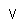button of the Schematic Editor's Toolbar. When the two inputs are zero or at least one of them is zero, the output voltage is 0V. When both inputs are high, the AND gate's output voltage jumps to +5V and VOUT = 2.5V as can be seen from the figures below.The output voltage of the AND gate when both digital inputs are low.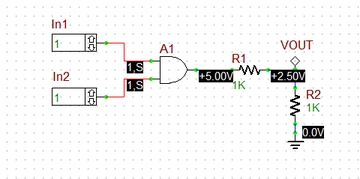The output voltage of the AND gate when both digital inputs are high.

## Testing a Mixed-Mode Circuit with Conversion Bridges

The following is a list of parts needed for this part of the tutorial lesson:

Part Name Part Type Part Value
In1 Digital Input 1-bit
In2 Digital Input 1-bit
Out1 Digital Output N/A
A1 Generic AND Gate Defaults
A2 Generic OR Gate Defaults
A3 - A4 1-Bit DAC Bridge Defaults, out_high = 3.3
A5 1-Bit ADC Bridge Defaults, in_high = 3
R1 - R2 Resistor 1k
R3 - R4 Resistor 2k
R5 - R6 Resistor 1k
Vcc DC Bias Voltage Source 15V
Vee DC Bias Voltage Source -15V
X1 LM741 Op-Amp Defaults

RF.Spice A/D also provides explicit A/D and D/A bridges for conversion between analog and digital signals and vice versa. Using an A/D bridge you can explicitly set the high and low input voltage levels for the input analog signal. Using a D/A bridge you can explicitly set the high and low output voltage levels for the output analog signal. These explicit values will override RF.Spice's default conversion voltage levels set in the SPICE Simulation Options Dialog. Place and connect the parts as shown in the figure below. Vcc and Vee are convenient DC bias voltage sources that you can use in conjunction with Op-Amp devices. Their default values are 5V and -5V, respectively. For this project, you need to change their values to 15V and -15V, respectively.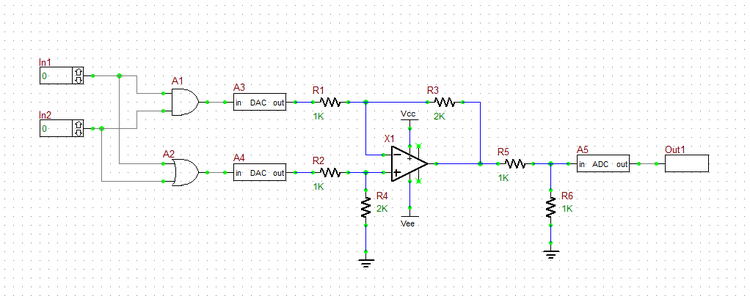A mixed-mode circuit containing AND and OR gates, an Op-Amp, and DAC and ADC conversion bridges.

Open the property dialog of the DAC bridge and change the value of the "out_high" parameter to 3.3V. Similarly, change the value of the "in-high" parameter to 3V in the property dialog of the ADC bridge. Note that when you change the properties of the DAC bridge A3, the properties of the DCA bridge A4 change accordingly.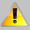When you change the properties of a shared model, all the parts sharing that model will be updated accordingly. To exclude a particular part from other similar parts and make it distinct, you should click the button labeled Make Model Unique.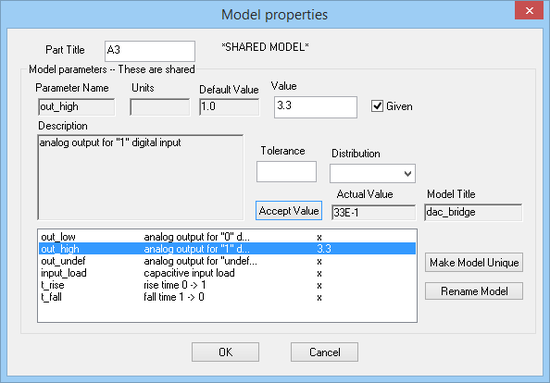The property dialog of the 1-bit DAC bridge device.The property dialog of the 1-bit ADC bridge device.

The DAC bridges at the output of the logic gates A1 and A2 produce an analog voltage of 3.3V when the output of the logic gate is at high or "1" state. Let's denote the output voltages of the two DAC bridges A3 and A4 by vi1 and vi2, respectively, denote the output voltage of the Op-Amp X1 by vo1, and denote the input voltage of the ADC bridge A5 by vo2. The Op-Amp circuit is a difference amplifier that you already explored in Analog Tutorial Lesson 6. The resistors R5 and R6 form a simple voltage divider. The following relationship holds:

$V_{o1} = \frac{ R_3 }{ R_1} \left( V_{i2} - V_{i1} \right) = 2 \left( V_{i2} - V_{i1} \right)$

$V_{o2} = \frac{ R_6 }{ R_5 + R_6} V_{o1} = \frac{1}{2} V_{o1} = V_{i2} - V_{i1}$

From these equations we get the following truth table, which is identical to that of an XOR gate:

In1 In2 vi1 vi2 vo1 vo2 Out
0 0 0V 0V 0V 0V 0
0 1 0V 3.3V 6.6V 3.3V 1
1 0 0V 3.3V 6.6V 3.3V 1
1 1 3.3V 3.3V 0V 0V 0

Run a live simulation of this circuit and enable circuit animation. Change the values of the two digital inputs and verify the above truth table. The figures below show some of these states: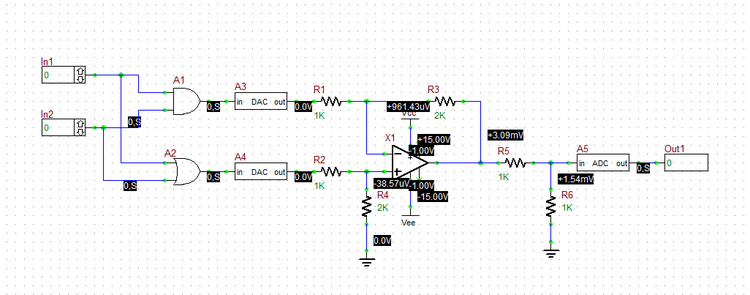The state of the mixed-mode circuits when In1 = In2 = 0.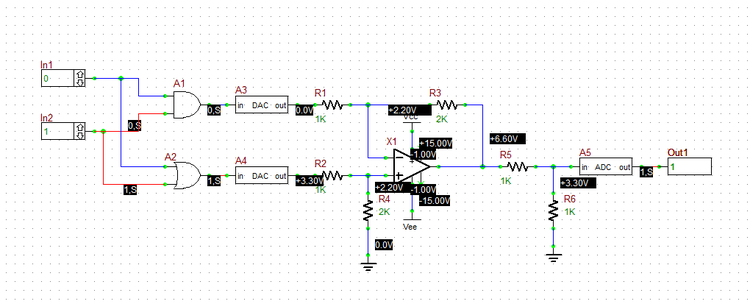The state of the mixed-mode circuits when In1 = 0 and In2 = 1.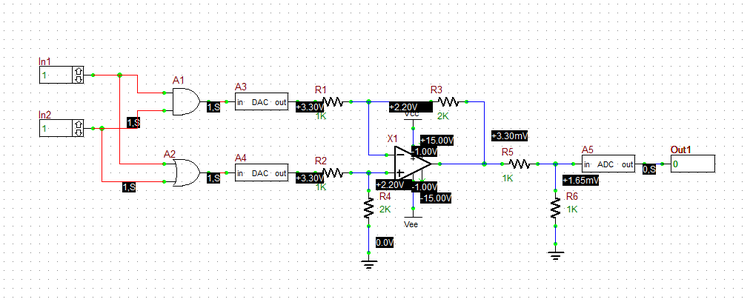The state of the mixed-mode circuits when In1 = In2 = 1.